xTriplet stateEncyclopedia
A spin triplet is a set of three quantum states of a system, each with total spin S = 1 (in units of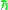). The system could consist of a single elementary massive spin 1 particle such as a W or Z boson, or be some multiparticle state with total spin angular momentum of one.

In physics
Physics
Physics is a natural science that involves the study of matter and its motion through spacetime, along with related concepts such as energy and force. More broadly, it is the general analysis of nature, conducted in order to understand how the universe behaves.Physics is one of the oldest academic...

, spin
Spin (physics)
In quantum mechanics and particle physics, spin is a fundamental characteristic property of elementary particles, composite particles , and atomic nuclei.It is worth noting that the intrinsic property of subatomic particles called spin and discussed in this article, is related in some small ways,...

is the angular momentum
Angular momentum
In physics, angular momentum, moment of momentum, or rotational momentum is a conserved vector quantity that can be used to describe the overall state of a physical system...

intrinsic to a body, as opposed to orbital angular momentum, which is the motion of its center of mass
Center of mass
In physics, the center of mass or barycenter of a system is the average location of all of its mass. In the case of a rigid body, the position of the center of mass is fixed in relation to the body...

about an external point. In quantum mechanics
Quantum mechanics
Quantum mechanics, also known as quantum physics or quantum theory, is a branch of physics providing a mathematical description of much of the dual particle-like and wave-like behavior and interactions of energy and matter. It departs from classical mechanics primarily at the atomic and subatomic...

, spin is particularly important for systems at atomic length scales, such as individual atoms, protons, or electrons. Such particles and the spins of quantum mechanical systems ("particle spin") possess several unusual or non-classical features, and for such systems, spin angular momentum cannot be associated with rotation but instead refers only to the presence of angular momentum.

## Two spin-1/2 particles

In a system with two spin-1/2 particles - for example the proton and electron in the ground state of hydrogen, measured on a given axis, each particle can be either spin up or spin down so the system has four basis states in all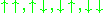using the single particle spins to label the basis states, where the first and second arrow in each combination indicate the spin direction of the first and second particle respectively.

More rigorously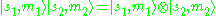and since for spin-1/2 particles, the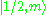basis states span a 2-dimensional space, the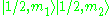basis states span a 4-dimensional space.

Now the total spin and its projection onto the previously defined axis can be computed using the rules for adding angular momentum in quantum mechanics
Quantum mechanics
Quantum mechanics, also known as quantum physics or quantum theory, is a branch of physics providing a mathematical description of much of the dual particle-like and wave-like behavior and interactions of energy and matter. It departs from classical mechanics primarily at the atomic and subatomic...

using the Clebsch–Gordan coefficients. In general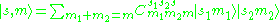substituting in the four basis states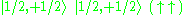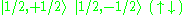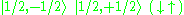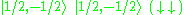returns the possible values for total spin given along with their representation in the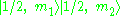basis. There are three states with total angular momentum 1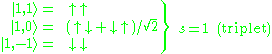and a fourth with total angular momentum 0.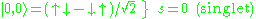The result is that a combination of two spin-1/2 particles can carry a total spin of 1 or 0, depending on whether they occupy a triplet or singlet state.

• Singlet state
• Doublet state
A diradical in organic chemistry is a molecular species with two electrons occupying two degenerate molecular orbitals . They are known by their higher reactivities and shorter lifetimes. In a broader definition diradicals are even-electron molecules that have one bond less than the number...

• Angular momentum
Angular momentum
In physics, angular momentum, moment of momentum, or rotational momentum is a conserved vector quantity that can be used to describe the overall state of a physical system...

• Pauli matrices
Pauli matrices
The Pauli matrices are a set of three 2 × 2 complex matrices which are Hermitian and unitary. Usually indicated by the Greek letter "sigma" , they are occasionally denoted with a "tau" when used in connection with isospin symmetries...

• Spin multiplicity
• Spin quantum number
Spin quantum number
In atomic physics, the spin quantum number is a quantum number that parameterizes the intrinsic angular momentum of a given particle...

• Spin-1/2
• Spin tensor
• Spinor
Spinor
In mathematics and physics, in particular in the theory of the orthogonal groups , spinors are elements of a complex vector space introduced to expand the notion of spatial vector. Unlike tensors, the space of spinors cannot be built up in a unique and natural way from spatial vectors...